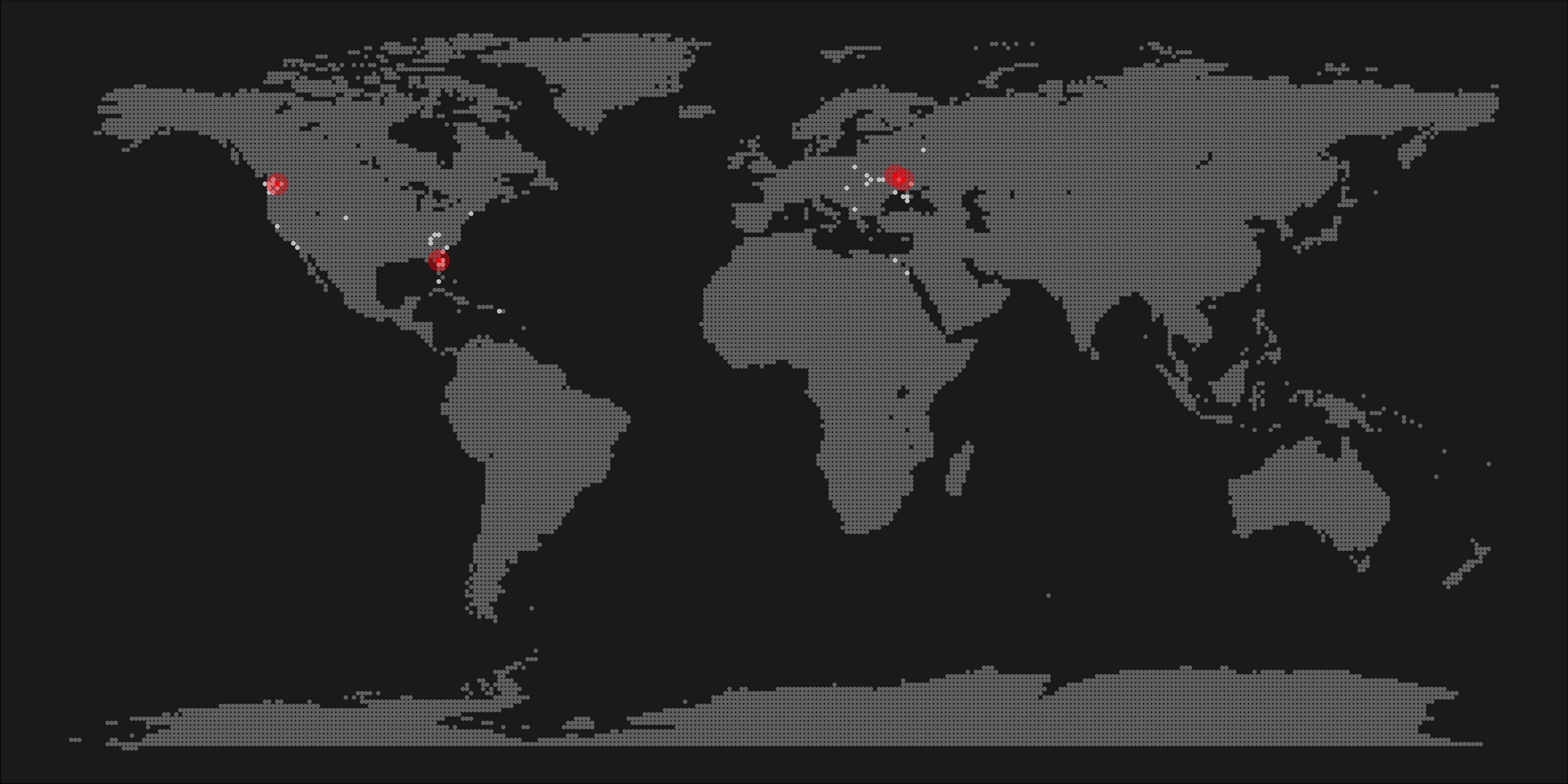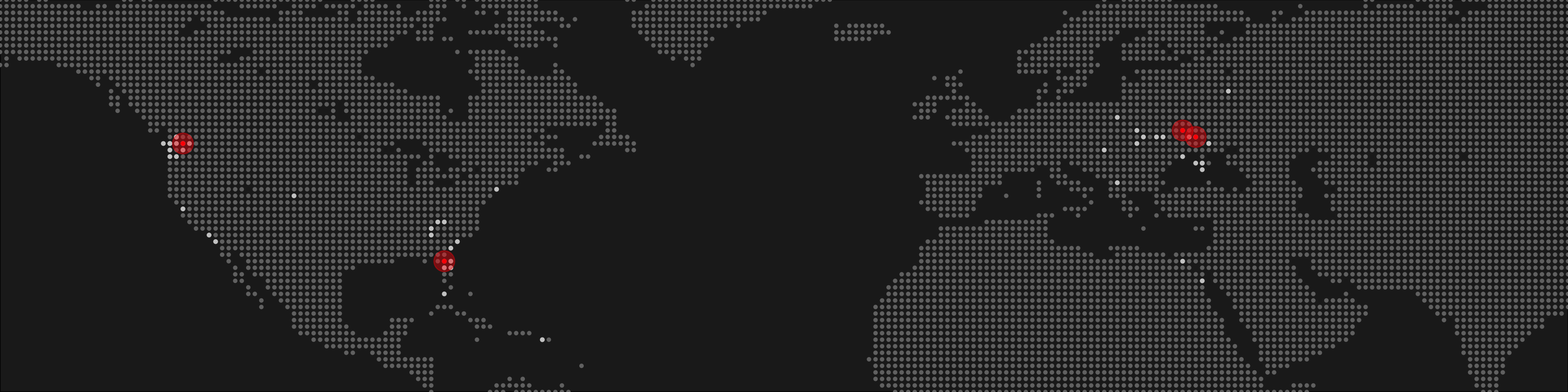Create World Pixel Maps in R

Posted on
ggplot ggmap geospatial maps r

*Cross-posted: Medium*

Today, I’m going to show you how to make pixel maps in R. Why pixel maps? Because they look awesome!

I was searching on the web for a while, but couldn’t find a good tutorial. Being stubborn as I am, I eventually figured out a way to get what I want. You know, if you torture your code enough, it might give you what you need.

Setup

First, of course, loading required packages. These days, I don’t bother with discrete packages and get the entire `tidyverse` right away. Aside from that, you may need the `ggmap` package, which I used in the earlier iterations of this script (more on that later). You’ll also need the `maps` package.

``````# Library -----------------------------------------------------------------

library(tidyverse)
library(maps)
library(here)``````

Next, we’ll need our data points. You can do anything you want here: load a google sheet with data, reach to you Google Maps data, import a csv file, whatever your heart desires.

Initially, I created a data frame with places I’ve been to, and then grabbed their coordinates with `mutate_geocode()` function from a `ggmap` package. That piece of code takes a while to run, and the list doesn’t really change that much, so I ended up saving it as a separate Google sheet, and now I simply import it. But you do as you wish.

You’ll obviously need to replace this chunk with your own data. I include `head` of my tibble to give you an idea about the data structure.

``````## Your location import method goes here.

```# A tibble: 10 x 4
city                    status    lon   lat
<chr>                   <chr>   <dbl> <dbl>
1 Anacortes, WA           been   -123.   48.5
2 Asheville, NC           been    -82.6  35.6
3 Atlanta, GA             been    -84.4  33.7
4 Boone, NC               been    -81.7  36.2
5 Budapest, Hungary       been     19.0  47.5
6 Cairo, Egypt            been     31.2  30.0
7 Canon Beach, OR         been   -124.   45.9
8 Charleston, SC          been    -79.9  32.8
9 Denver, CO              been   -105.   39.7
10 Dnipropetrivsk, Ukraine been     35.0  48.5```

Rounding the coordinates

As I’m creating a pixel map - I need dots in the right places. I’m going to plot a dot for each degree, and therefore I need my coordinates rounded to the nearest degree

``````locations <- locations %>%
mutate(long_round = round(lon, 0),
lat_round = round(lat,0))
tail(locations)``````

Generate a pixel grid

The next step is the key to getting a pixel map. We’ll fill the entire plot with a grid of dots - 180 dots from south to north, and 360 dots from east to west, but then only keep the dots that are on land. Simple!

``````# Generate a data frame with all dots -----------------------------------------------

lat <- data_frame(lat = seq(-90, 90, by = 1))
long <- data_frame(long = seq(-180, 180, by = 1))
dots <- lat %>%
merge(long, all = TRUE)

## Only include dots that are within borders. Also, exclude lakes.
dots <- dots %>%
mutate(country = map.where('world', long, lat),
lakes = map.where('lakes', long, lat)) %>%
filter(!is.na(country) & is.na(lakes)) %>%
select(-lakes)

Plot

And now the easy part. Plotting.

Please note that this post, just like this entire site, runs on `blogdown`, and the post is created via Rmarkdown. When the plots render here - they look ugly-ish due to the fact that geom_point doesn’t scale down along with the plot. The output on your machine will look better. Take a look at the head image to understand how your output may look like

``````theme <- theme_void() +
theme(panel.background = element_rect(fill="#212121"),
plot.background = element_rect(fill="#212121"),
plot.title=element_text(face="bold", colour="#3C3C3C",size=16),
plot.subtitle=element_text(colour="#3C3C3C",size=12),
plot.caption = element_text(colour="#3C3C3C",size=10),
plot.margin = unit(c(0, 0, 0, 0), "cm"))

plot <- ggplot() +
#base layer of map dots
geom_point(data = dots, aes(x=long, y = lat), col = "grey45", size = 0.7) +
#plot all the places I've been to
geom_point(data = locations, aes(x=long_round, y=lat_round), color="grey80", size=0.8) +
#plot all the places I lived in, using red
geom_point(data = locations %>% filter(status == 'lived'), aes(x=long_round, y=lat_round), color="red", size=0.8) +
#an extra layer of halo around the places I lived in
geom_point(data = locations %>% filter(status == 'lived'), aes(x=long_round, y=lat_round), color="red", size=6, alpha = 0.4) +
theme
plot``````Looking at the map of the entire world can be overwhelming and sad, especially if you, just like me, are not much of a traveler. Look at it! There aren’t many dots! WTF?! Sad!

You can zoom in on an area you did cover (e.g. include USA only), either computationally (calculate you westernmost, easternmost, southernmost and northernmost points and pass them as xlim and ylim), or excluding continents from the map with `dplyr` (excluding Antarctica at least would be a good idea). You can also use a different map to start with - World map may not be necessary for some tasks. I used it because I was fortunate enough to live on 2 continents, but your mileage may vary.

In all of these cases, you may want to reconsider the grain of the map: if you zoom in on USA only, you may want to choose to plot a dot for every 0.5 degrees, and then would need to adjust your coordinate rounding respectively (round to the nearest half of degree). Why do it? The finer your grain - the more details you’ll get. For instance, with a grain of 1 degree, San Francisco, San Mateo, San Rafael and Oakland are all be one same dot.

I could definitely program my way though this scaling issue and create a parameter, and make other variables depend on it… I don’t find this exercise to be particularly useful in this case. If you get it done - awesome!

For my case, I wanted a wide banner, so I chose some specific arbitrary limits that looked good to me.

``````plot + scale_y_continuous(limits = c(10, 70), expand = c(0,0)) +
scale_x_continuous(limits = c(-150,90), expand = c(0,0))``````Outro

Obviously, there is so much more to do with this. The possibilities are endless. The basic idea is pretty simple - generate a point grid and plot rounded coordinates on top of the grid.

Let me know if you find new implementations of this code!